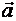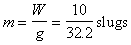Notation:

 Book By hand Scalar a a Vector a  (bold faced) a  orUnits:

 SI U.S. Mass M kilogram kg slug Length L meter m feet ft Force F Newton N pound lb Time T second s second sec

Relation between units is based on the equation F=ma:

1 N = (1 kg) (1 m/s2)

1 lb= (1 slug) (1 ft/sec2 )

 Example of calculating mass in U.S. system: The mass, m, of a particle which weighs W=10 lb and is in a gravitational field of with an acceleration of gravity g=32.2 ft/sec2 isUnit conversion:

 l lb = 4.4482 N 1 slug = 14.5938 kg 1 ft = 0.3048 m 1 ft = 12 in 1 mile = 5,280 ft 1 kip = 1,000 lb 1 ton = 2,000 lb

Rounding numbers:Check equations by checking that all terms in an equation have the same dimensions